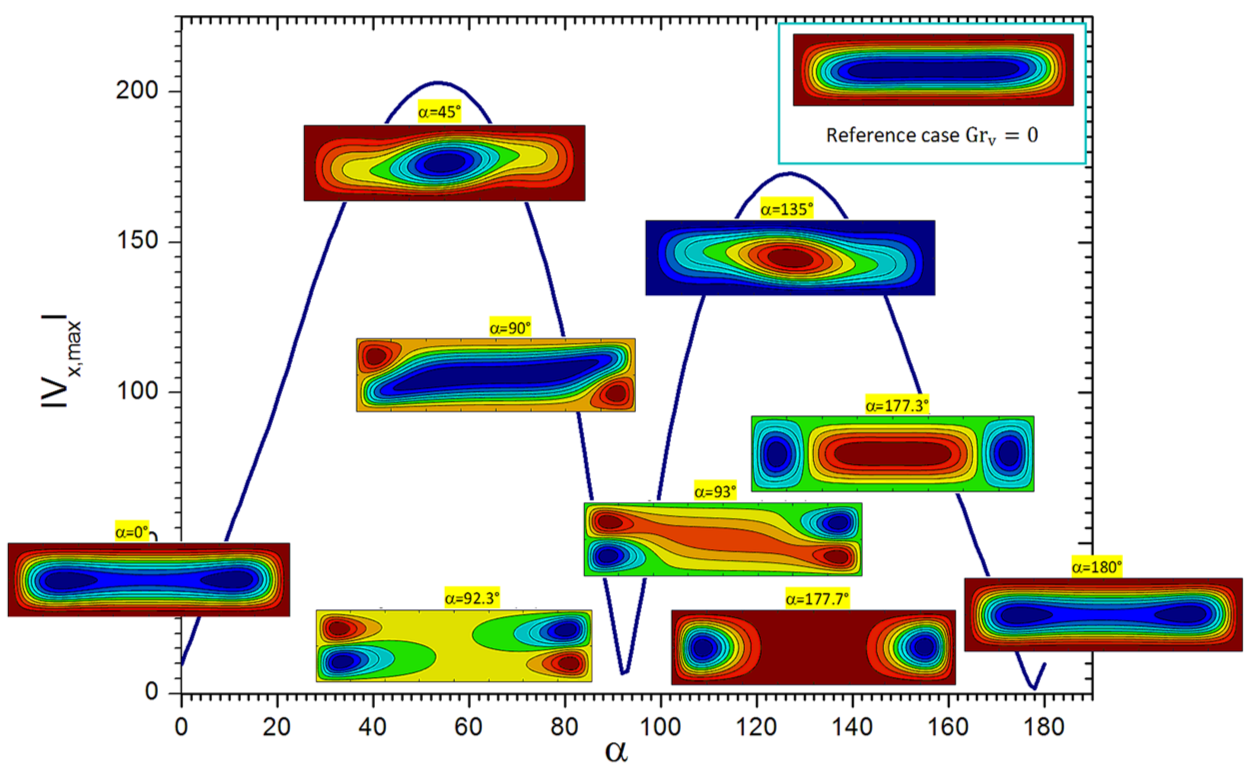# Laboratoire de Mécanique des Fluides et d’Acoustique - UMR 5509

LMFA - UMR 5509
Laboratoire de Mécanique des Fluides et d’Acoustique
Lyon
France

Article dans Phys. Fluids (2019)

## Theoretical and numerical study on high frequency vibrational convection : Influence of the vibration direction on the flow structure

Samia Bouarab, Faiza Mokhtari, Slim Kaddeche, Daniel Henry, Valéry Botton & Abdessamed Medelfef

Thermal convection induced simultaneously by horizontal temperature gradient and vibration in a rectangular cavity filled with molten silicon is investigated numerically and theoretically. The time averaged equations of convection are solved in the high-frequency vibration approximation. The Chebyshev spectral collocation method and a Newton-type method based on the Frechet derivative are used in the numerical solution of the streamfunction formulation of the incompressible Navier-Stokes equations. Validation by comparison with previous studies has been performed. Different values of the Grashof number $\mathrm{Gr}$ and vibrational Grashof number $\mathrm{Gr}_v$ and all the possible orientations of the vibrations are considered. Numerical results show that depending on the vibration direction, the flow can be amplified or damped, with even the possibility of flow inversion which can occur between critical vibration angles $\alpha_1$ and $\alpha_2$. A general theoretical expression is derived relating these critical angles and the ratio of vibrational to buoyant convection parameters, $\mathrm{Gr}_v /\mathrm{Gr}$. A very good agreement between the theoretical and numerical results is obtained.